• 等差数列是指从第二项起，每一项与它的前一项的差等于同一个常数的一种数列，常用A、P表示。这个常数叫做等差数列的公差。前n项和公式为：Sn=a1*n+[n*(n-1)*d]/2或Sn=[n*(a1+an)]/2。等差数列公式1.定义式2.通项公式...

等差数列是指从第二项起，每一项与它的前一项的差等于同一个常数的一种数列，常用A、P表示。这个常数叫做等差数列的公差。前n项和公式为：Sn=a1*n+[n*(n-1)*d]/2或Sn=[n*(a1+an)]/2。等差数列公式

1.定义式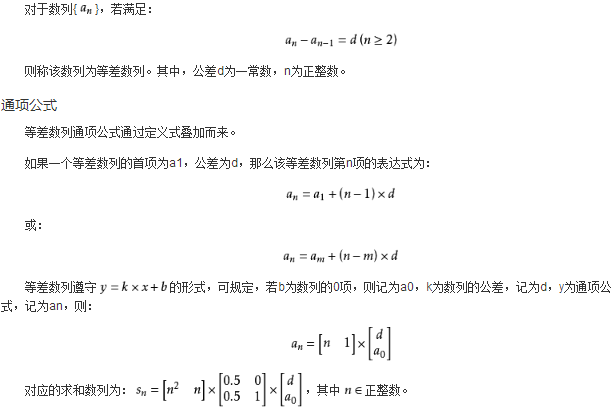2.通项公式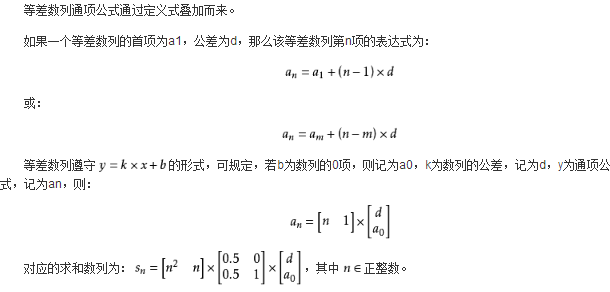3.求和公式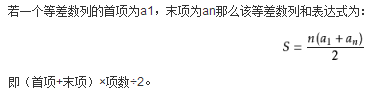4.前n项和公式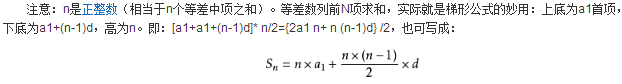等差数列推论

(1)从通项公式可以看出，a(n)是n的一次函数(d≠0)或常数函数(d=0)，(n，an)排在一条直线上，由前n项和公式知，S(n)是n的二次函数(d≠0)或一次函数(d=0，a1≠0)，且常数项为0。

(2)从等差数列的定义、通项公式，前n项和公式还可推出：a(1)+a(n)=a(2)+a(n-1)=a(3)+a(n-2)=…=a(k)+a(n-k+1)，(类似：p(1)+p(n)=p(2)+p(n-1)=p(3)+p(n-2)=。。。=p(k)+p(n-k+1))，k∈{1,2,…,n}。

(3)若m，n，p，q∈N*，且m+n=p+q，则有a(m)+a(n)=a(p)+a(q)，S(2n-1)=(2n-1)*a(n)，S(2n+1)=(2n+1)*a(n+1)，S(k)，S(2k)-S(k)，S(3k)-S(2k)，…，S(n)*k-S(n-1)*k…成等差数列，等等。若m+n=2p，则a(m)+a(n)=2*a(p)。

证明：p(m)+p(n)=b(0)+b(1)*m+b(0)+b(1)*n=2*b(0)+b(1)*(m+n)；p(p)+p(q)=b(0)+b(1)*p+b(0)+b(1)*q=2*b(0)+b(1)*(p+q)；因为m+n=p+q，所以p(m)+p(n)=p(p)+p。

(4)其他推论：

①和=(首项+末项)×项数÷2；

②项数=(末项-首项)÷公差+1；

③首项=2x和÷项数-末项或末项-公差×(项数-1)；

④末项=2x和÷项数-首项；

⑤末项=首项+(项数-1)×公差；

⑥2(前2n项和-前n项和)=前n项和+前3n项和-前2n项和。

数列求和方法

1、公式法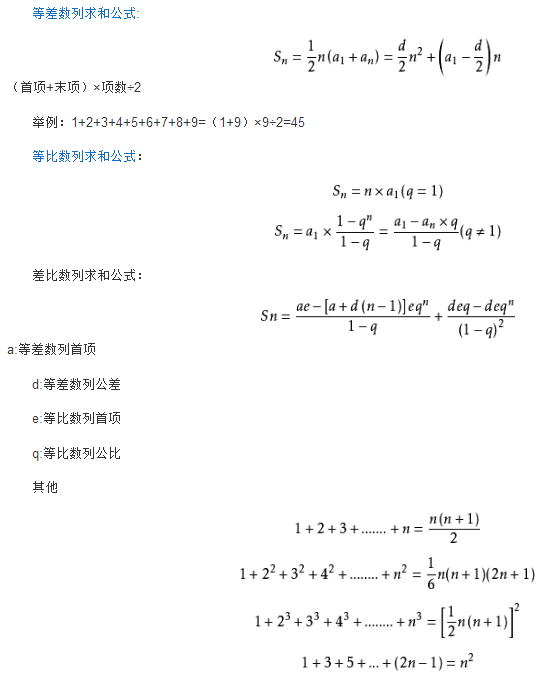2、错位相减法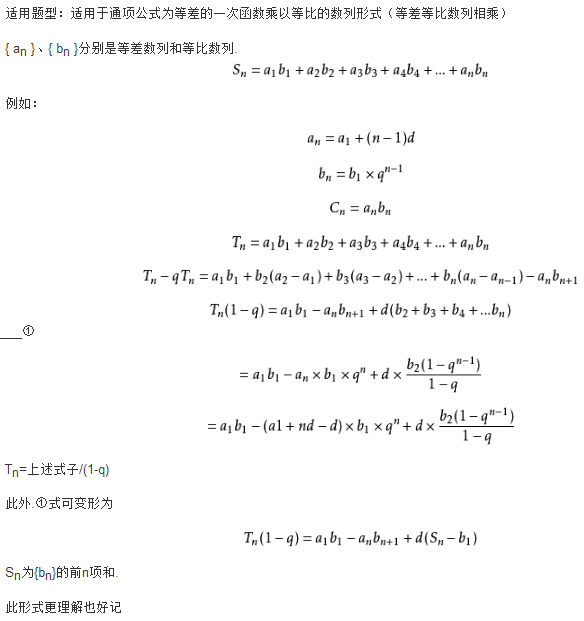3、倒序相加法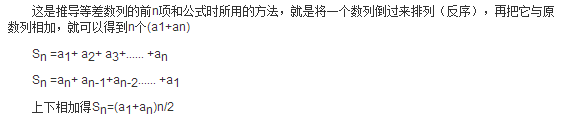4、分组法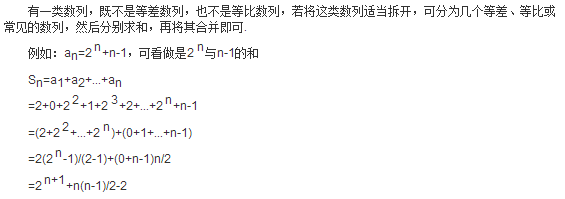5、裂项相消法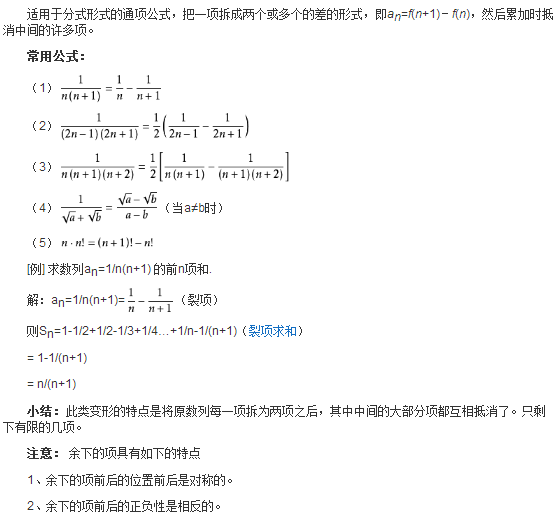6、数学归纳法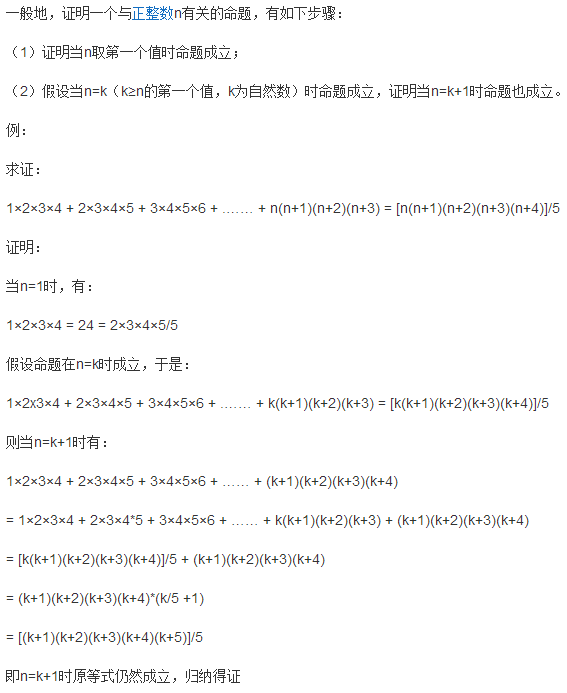7、通项化归法

先将通项公式进行化简，再进行求和。

如：求数列1，1+2，1+2+3，1+2+3+4,……的前n项和。此时先将an求出，再利用分组等方法求和。

8、并项求和法

(常采用先试探后求和的方法)

例：1－2+3－4+5－6+……+(2n-1)-2n

方法一：(并项)

求出奇数项和偶数项的和，再相减。

方法二：

(1－2)+(3－4)+(5－6)+……+[(2n-1)-2n]

方法三：

构造新的数列，可借用等差数列与等比数列的复合。

an=n(-1)^(n+1)

9、求和公式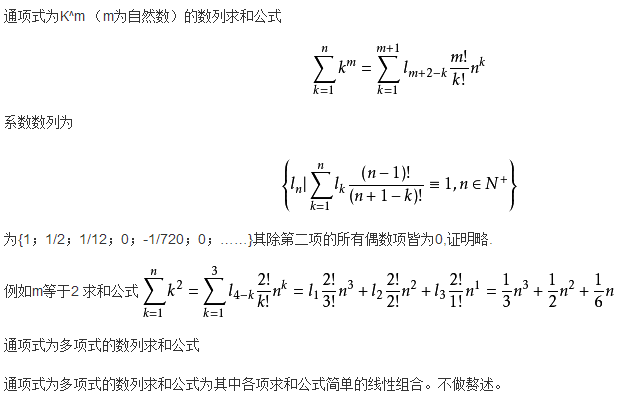展开全文• 等差数列求和公式

万次阅读 2018-10-08 11:09:22
等差数列是常见数列的一种，可以用AP表示，如果一个数列从第二项起，每一项与它的前一项的差等于同一个常数，这个数列就叫做等差数列，而这个常数叫做等差数列的公差，公差常用字母d表示。例如：1,3,5,7,9……（2n-1...

等差数列是常见数列的一种，可以用AP表示，如果一个数列从第二项起，每一项与它的前一项的差等于同一个常数，这个数列就叫做等差数列，而这个常数叫做等差数列的公差，公差常用字母d表示。例如：1,3,5,7,9……（2n-1)。等差数列{an}的通项公式为：an=a1+(n-1)d。前n项和公式为：Sn=n*a1+n(n-1)d/2或Sn=n(a1+an)/2。注意： 以上n均属于正整数

1. 一般定义
2. 扩展:幂次数列
3. 其他结论
1. 特殊性质
2. 求和公式（字母）
1. 求和公式（文字）

一般定义

编辑

等差数列遵守的形式,

可规定b为数列的0项,记为a0,k为数列的公差,记为d,y为通项公式,记为an对应的求和数列其中正整数

扩展:幂次数列

编辑

数列:求和数列:方阵等差数列是幂次数列的特殊形式

数列:求和数列:其他结论

编辑

首项：/末项-(项数-1)×公差

末项：通项公式：项数：公差：如：数列1,3,5,7,……,97,99 公差就是d=3-1=2 将推广到，则为：a1,a2,a3....an,n=奇数，Sn=（a（（n-1）/2)）*((n-1）/2)

特殊性质

编辑

1.在数列中,若,则有:

①若,则am+an=ap+aq.

②若m+n=2q,则am+an=2aq.

2.在等差数列中，若Sn为该数列的前n项和，S2n为该数列的前2n项和，S3n为该数列的前3n项和，则Sn，S2n-Sn，S3n-S2n也为等差数列。

求和公式（字母）

编辑

设首项为, 末项为, 项数为, 公差为, 前项和为, 则有:

①;

②;

③;

④, 其中..

当d≠0时，Sn是n的二次函数，（n，Sn）是二次函数的图象上一群孤立的点。利用其几何意义可求前n项和Sn的最值。

注意：公式一二三事实上是等价的，在公式一中不必要求公差等于一。

求和推导

证明：由题意得：

Sn=a1+a2+a3+。。。+an①

Sn=an+a（n-1）+a（n-2）+。。。+a1②

①+②得：

2Sn=[a1+an]+[a2+a(n-1)]+[a3+a(n-2)]+...+[a1+an](当n为偶数时)

Sn={[a1+an]+[a2+a(n-1)]+[a3+a(n-2)]+...+[a1+an]}/2

Sn=n（A1+An）/2 (a1，an，可以用a1+（n-1）d这种形式表示可以发现括号里面的数都是一个定值，即A1+An）

求和公式（文字）

编辑

【（首项+末项）×项数】÷2

首项×项数+【项数（项数-1）×公差】/2

{【2首项+（项数-1）×公差】项数}/2

展开全文等差数列求和
• 等差数列求和公式1.公式法2.错位相减法3.求和公式4.分组法有一类数列，既不是等差数列，也不是等比数列，若将这类数列适当拆开，可分为几个等差、等比或常见的数列，然后分别求和，再将其合并即可.5.裂项相消法适用...

数学大师

等差数列是常见数列的一种，可以用AP表示，如果一个数列从第二项起，每一项与它的前一项的差等于同一个常数，这个数列就叫做等差数列，而这个常数叫做等差数列的公差，公差常用字母d表示。

1.公式法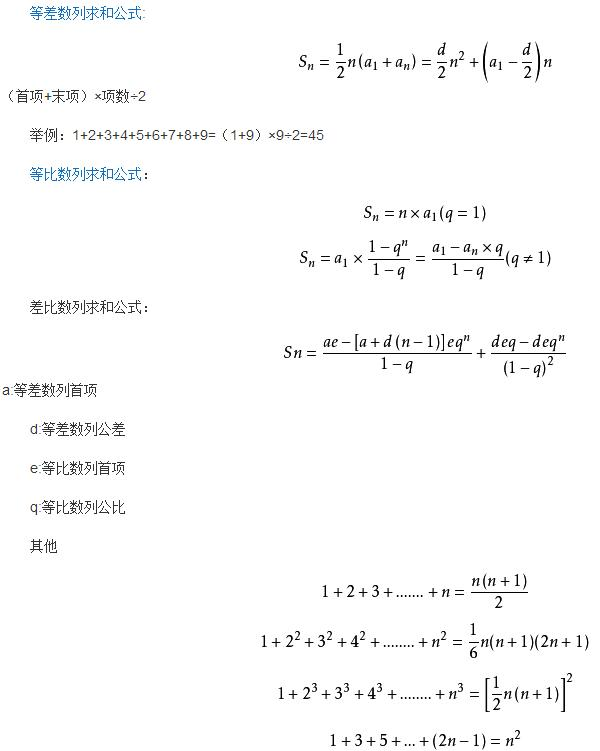2.错位相减法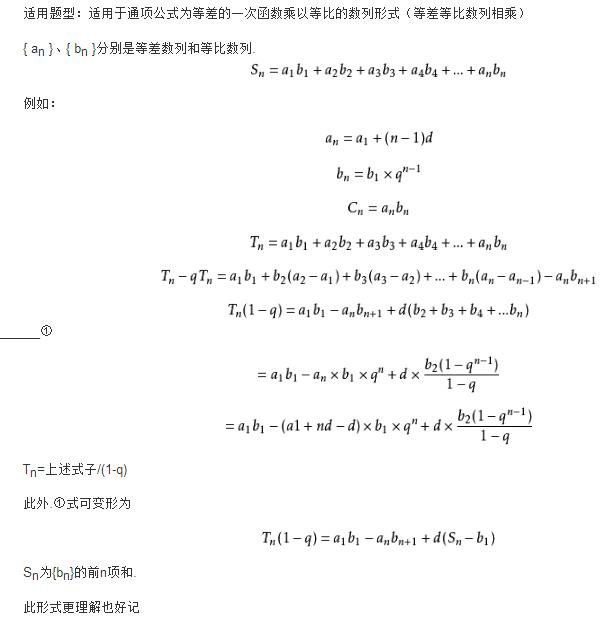3.求和公式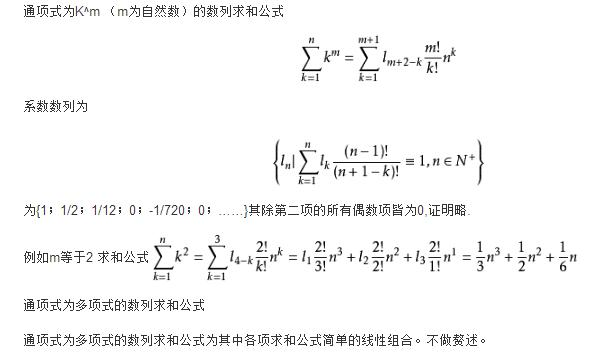4.分组法

有一类数列，既不是等差数列，也不是等比数列，若将这类数列适当拆开，可分为几个等差、等比或常见的数列，然后分别求和，再将其合并即可.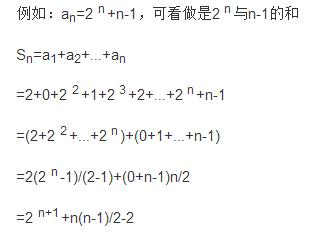5.裂项相消法

适用于分式形式的通项公式，把一项拆成两个或多个的差的形式，即an=f(n+1)－f(n)，然后累加时抵消中间的许多项。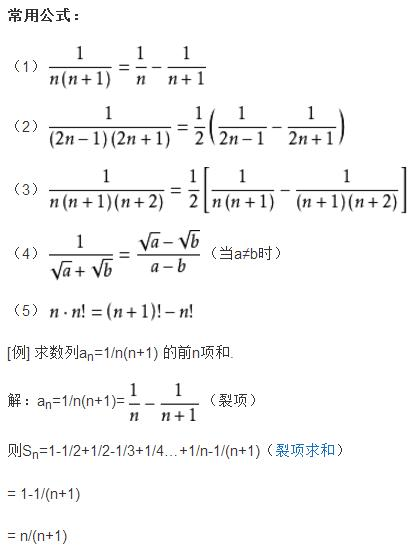【小结】此类变形的特点是将原数列每一项拆为两项之后，其中中间的大部分项都互相抵消了。只剩下有限的几项。
注意：余下的项具有如下的特点1、余下的项前后的位置前后是对称的。2、余下的项前后的正负性是相反的。

6.数学归纳法

一般地，证明一个与正整数n有关的命题，有如下步骤：(1)证明当n取第一个值时命题成立；(2)假设当n=k(k≥n的第一个值，k为自然数)时命题成立，证明当n=k+1时命题也成立。

【例】求证：1×2×3×4 + 2×3×4×5 + 3×4×5×6 + .…… + n(n+1)(n+2)(n+3) = [n(n+1)(n+2)(n+3)(n+4)]/5

证明：当n=1时，有：1×2×3×4 = 24 = 2×3×4×5/5

假设命题在n=k时成立，

于是：1×2x3×4 + 2×3×4×5 + 3×4×5×6 + .…… + k(k+1)(k+2)(k+3) = [k(k+1)(k+2)(k+3)(k+4)]/5

则当n=k+1时有：

1×2×3×4 + 2×3×4×5 + 3×4×5×6 + …… + (k+1)(k+2)(k+3)(k+4)

= 1×2×3×4 + 2×3×4*5 + 3×4×5×6 + …… + k(k+1)(k+2)(k+3) + (k+1)(k+2)(k+3)(k+4)

= [k(k+1)(k+2)(k+3)(k+4)]/5 + (k+1)(k+2)(k+3)(k+4)

= (k+1)(k+2)(k+3)(k+4)*(k/5 +1)

= [(k+1)(k+2)(k+3)(k+4)(k+5)]/5

即n=k+1时原等式仍然成立，归纳得证

7.并项求和法

(常采用先试探后求和的方法)【例】1－2+3－4+5－6+……+(2n-1)-2n

方法一：(并项)求出奇数项和偶数项的和，再相减。

方法二：(1－2)+(3－4)+(5－6)+……+[(2n-1)-2n]

方法三：构造新的数列，可借用等差数列与等比数列的复合。an=n(-1)^(n+1)

等差数列判定及其性质

等差数列的判定
(1)a(n+1)--a(n)=d (d为常数、n ∈N*)[或a(n)--a(n-1)=d，n ∈N*，n ≥2,d是常数]等价于{a(n)}成等差数列。
(2)2a(n+1)=a(n)+a(n+2) [n∈N*] 等价于{a(n)}成等差数列。
(3)a(n)=kn+b [k、b为常数,n∈N*] 等价于{a(n)}成等差数列。
(4)S(n)=A(n)^2 +B(n) [A、B为常数，A不为0，n ∈N* ]等价于{a(n)}为等差数列。

特殊性质
在有穷等差数列中，与首末两项距离相等的两项和相等。并且等于首末两项之和；特别的，若项数为奇数，还等于中间项的2倍,即，a(1)+a(n)=a(2)+a(n-1)=a(3)+a(n-2)=···=2*a中

【例】数列：1，3，5，7，9，11中

a(1)+a(6)=12 ;

a(2)+a(5)=12 ;

a(3)+a(4)=12 ;

即，在有穷等差数列中，与首末两项距离相等的两项和相等。并且等于首末两项之和。

数列：1，3，5，7，9中

a(1)+a(5)=10 ;

a(2)+a(4)=10 ;

a(3)=5=[a(1)+a(5)]/2=[a(2)+a(4)]/2=10/2=5 ;

即，若项数为奇数，和等于中间项的2倍，另见，等差中项。

​数学大师

展开全文无穷级数求和7个公式
• 下半年的事业单位考试正在来袭，在大家备考过程中不难发现，计算问题属于一类高频考点，而计算问题中的等差数列又是这一类题里最常考的知识点，那么，今天和大家分享的是等差数列中中项法求和公...

【导读】

中公事业单位为帮助各位考生顺利通过事业单位招聘考试！今天为大家带来数量关系：等差数列中项法求和的应用。

【导读】

中公事业单位为帮助各位考生顺利通过事业单位招聘考试！今天为大家带来数量关系：等差数列中项法求和的应用。

下半年的事业单位考试正在来袭，在大家备考过程中不难发现，计算问题属于一类高频考点，而计算问题中的等差数列又是这一类题里最常考的知识点，那么，今天和大家分享的是等差数列中中项法求和公式的应用。我们初中时就知道了等差数列的通项公式和求和公式，但是那时主要应用的求和公式是：前n项和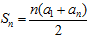，即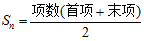，而在实际解题过程中我们发现，等差数列的中项法求和公式应用起来解题会更快。所以，对于等差数列的学习，一定要掌握中项法求和的方式。

中项法求和分为两种情况，一是数列为奇数项时：Sn=中间一项×项数。

【例1】主席台前排坐着5个人，最小的一个32岁，从第二个起，每个人都比前一个人年龄大3岁，则这个5个人的平均年龄为( )

A.28 B.35 C.38 D.41

【答案】C。

【中公解析】方法一，依题意可知，5人年龄构成公差为3的等差数列，求5人的平均年龄，只需求5人的年龄和，再除以5即可，a1=32,根据通项公式易知a5=44，则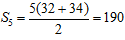，所以，5人平均年龄为190÷5=38。

方法二，由中项法求和可知：五个人的年龄和S5=第三个人的年龄×5，所以第三个人的年龄即等于5人的平均年龄，第一人是32岁，则第二人为35岁，第三人为38岁，此题选C。

中项法求和的另一种情况是数列为偶数项时：Sn=中间两项和×项数的一半。

【例2】一张试卷共8道题，后面每一道题总比前一道多4分，如果试卷满分120分，那么第四道题分值是：

A.17 B.16 C.13 D.11

【答案】C。

【中公解析】方法一，依题意，8道题的分值构成公差为4的等差数列，8项的和S8=120，根据通项公式和常规求和公式有：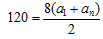……①;a8=a1+(8-1)×4……②;联立两式解得a1=1,所以，a4=1+(4-1)×4=13。

方法二，由中项法求和可知：S8=120=(a4+a5)×4，则(a4+a5)=30，又因为a5比a4大4，所以a5=17，a4=13.此题选C.

通过以上两道例题不难看出，在等差数列的计算问题中，如果能灵活运用中项法求和公式，那么解题过程也许会变得简单，更容易得到结果，所以，在事业单位备考过程中，一定要对中项法求和很熟悉，做到灵活运用。

相关推荐

展开全文• 题目链接 题意： 给你 [ L，R ] 一个区间，区间内...找出两个最前和最后的奇数和偶数，利用等差求和求得。 #include&lt;bits/stdc++.h&gt; using namespace std; typedef long long ll; int main() { ...
• 题：等差数列可以定义为每一项与它的前一项的差等于一个常数，可以用变量 x1 表示等差数列的第一项，用 d 表示公差，请计算数列1 4 7 10 13 16 19 …前 100 项的和。等差求和公示：和=(首数+尾数)*项数/2;题的懵就是...
• 就是很简单的一个数列求和问题，运用到n*(1+n)/2公式，答主在做题的时候还在想，是否会出现除法的问题，但是n*(1+n)一定是一个偶数(证明过程过于简单，就不在这里列举出来了)，所以最后求得的解一定是一个整数，所以...高斯算法
• 等差数列：2 5 8… 等差数列前n项和公式：sum= 在写编程时，第一次各项的顺序是（a1+an）/2*N，结果测试用例部分编译出现错误； 分析发现（a1+an）可能是奇数，所以直接除以2，会出现小数部分被忽略的情况，因此出现...算法
• 等差数列

2021-05-26 00:44:54
1354. 等差数列2021-05-24 20:06:01预处理出双平方数集合枚举双平方数中的一对数作为等差数列的首项和第二项剪枝：计算出当前等差数列的末项，last=x+(n-1)*d比双平方数集合中最大的数还要大，则无需判断其是否能...
• 一、等差数列的相关概念： 定义：自然语言，略；符号语言：$$a_n-a_{n-1}=d(n\ge 2，n\in N^*)$$，$$d$$为常数或$$a_{n+1}-a_n=d(n\in N^*)$$， 等差中项：若$$a，A，b$$成等差数列，则$$A$$称为$$a$$与$$b$$的等差...
• 《剑指Offer》Java刷题 NO.41 和为S的连续正数序列(序列数学规律、等差数列求和公式、边界条件、滑动窗口、动态规划) 传送门：《剑指Offer刷题总目录》 时间：2020-06-25 题目： 小明很喜欢数学,有一天他在做数学...
• 我们会惊奇的发现，奇数平方和和偶数平方和是一样的！ 此时一个同学提问到：按理说偶数项不是每项比奇数项多2*n+1
• n是偶数 平均值=S/n，且为偶数，平均值的小数部分是0.5.也就是(S%n)/n=0.5,所以条件是 sum%n*2==n 且根据S=（1+n）*n/2从1开始求出n的取值范围 n<根号2S import java.util.ArrayList; public class ...
• 题意：给出n, k，求分析：假设，则k mod (i+1) = k - (i+1)*p = k - i*p - p = k mod i - p则对于某个区间，i∈[l, r]，k/i的整数部分p相同，则其余数成等差数列，公差为-p然后我想到了做莫比乌斯反演时候有个分块...
• 很多同学抱怨平时花费大量时间，但数学成绩不见提升，该学了也学了，可是一考试发现还是不会做，其实在数学...(2016·高考北京卷)已知{an}是等差数列，{bn}是等比数列，且b2＝3，b3＝9，a1＝b1，a14＝b4. (1)求{an...高中数学
• 解题思路:使用数组来记录等差数列 然后分情况进行计算注意事项:参考代码:importjava.util.Scanner;publicclassMain{publicstaticvoidmain(String[]args){Scannerscanner=newScanner(System.in);while(scanner....
• labview初学者练习迭代次数求和小实例，以及提示对话框的使用labview
• 解题 都等于最中间的数就好了，剩余的数等差数列求和 （首项+末项）* 长度/2 class Solution { public: int minOperations(int n) { int an_1 = 2*(n-1)+1; int mid = (an_1+1)/2; if(n&1) return n/2*mid-(1+...
• 数列中分奇偶数项求和问题数列求和 中有一 复 的求和 ,要 正整数 n 行分奇数和偶数情形的, 例 明如下：一、相 两 符号相异；例 1：求和：n 1Sn? ( -1 )( 4n-3)n N解：当 n 偶数 ： Sn1 5 9 13nnnn4) n-12当 n 奇数 ...
• 等差数列是我们小学必须要掌握的基础知识，在小升初考试多以填空题、计算题的形式出现，并且在初、高中的学习过程中也会经常遇见它，现在就让我们一起来学习一下吧！知识点：1.把若干个数排成一列叫数列，其中每一个...
• 传送门 题意：  给你一个N*M大小的矩阵，表示一个“拼图”模型，这个拼图有N*M-1个块，现在有1~N*M-1个编号，给你一个P,每次取第1个，第1+P个，第1...原排列的写出来之后写成一排，这个序列的逆序数为偶数即“YES”,否
• 数学老师给小明出了一道等差数列求和的题目。但是粗心的小明忘记了一 部分的数列，只记得其中 N 个整数。 现在给出这 N 个整数，小明想知道包含这 N 个整数的最短的等差数列有 几项？ 【输入格式】 输入的第一行包含...c++
• 偶数求和

2019-05-02 19:28:32
=100)的数列，该数列定义为从2开始的递增有序偶数（公差为2的等差数列），现在要求你按照顺序每m个数求出一个平均值，如果最后不足m个，则以实际数量求平均值。编程输出该平均值序列。 输入 输入数据有多组，每组占...C语言 偶数求和
• 偶数求和 Problem Description 有一个长度为n(n<=100)的数列，该数列定义为从2开始的递增有序偶数，现在要求你按照顺序每m个数求出一个平均值，如果最后不足m个，则以实际数量求平均值。编程输出该平均值序列。 ...c语言 acm竞赛
• 本篇内容在知识地图的位置：数字：上图参考文本： 《数字起源 数的概念 从具体到抽象 奇数与偶数 质数 素数 13：虚数：虚构这个工具有什么用？ 催化剂、传声筒 时空连接 坐标系 14：无穷：如何理解无限大的世界？ ...无穷级数求和7个公式
• 序号：#31 难度：有挑战 时间限制：1000ms 内存限制：10M 描述 给出一个正整数N（2<... 其中 15 自身就是一个等差数列，3+5+7=15 也是一个符合条件的等差数列，所以输出为 2，表示有两个符合条件的等差数列。 请注...
• 【偶数求和】

2019-10-15 21:31:42
=100)的数列，该数列定义为从2开始的递增有序偶数（公差为2的等差数列），现在要求你按照顺序每m个数求出一个平均值，如果最后不足m个，则以实际数量求平均值。编程输出该平均值序列。 输入 输入数据有多组，每组占...C语言
• 题意：给出n，求一共有多少个连续的数满足加和恰好得到n， 思路：这题想了好久一...根据等差数列求和公式S=（a1+an）*n/2和末项公式an=a1+(n-1)*d(d位公差)得a1=(2*s+n-n*n)/2/n;得出求a1的公式然后对所有的n（...
• HDU2015 偶数求和【入门】

万次阅读 多人点赞 2016-06-27 08:00:13
偶数求和 Time Limit: 2000/1000 MS (Java/Others)Memory Limit: 65536/32768 K (Java/Others) Total Submission(s): 98886Accepted Submission(s): 41277 Problem Description 有一个长度为n(n InputHDU2015 偶数求和
• 有一个长度为n(n)的数列，该数列定义为从2开始的递增有序偶数，现在要求你按照顺序每m个数求出一个平均值，如果最后不足m个，则以实际数量求平均值。编程输出该平均值序列。   Input 输入数据有...ACM HDU 偶数求和...